By Learnbay Category Data Science Reading time 4-5 mins Published on Nov 25, 2021

## Data Preprocessing

Introduction to Data Preprocessing:- Before modeling the data we need to clean the information to get a training sample for the modeling. Data preprocessing is a data mining technique that involves transforming the raw data into an understandable format. It provides the technique for cleaning the data from the real world which is often incomplete, inconsistent, lacking accuracy and more likely to contain many errors. Preprocessing provides clean information before it gets to the modeling phase.

## Preprocessing of data in a stepwise fashion in scikit learn.

1. Introduction to Preprocessing:
• Learning algorithms have an affinity towards a certain pattern of data.
• Unscaled or unstandardized data have might have an unacceptable prediction.
• Learning algorithms understand the only number, converting text image to number is required.
• Preprocessing refers to transformation before feeding to Machine Learning.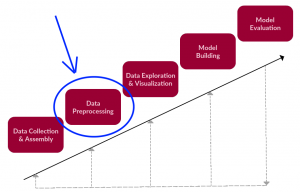1. StandardScaler
• The StandardScaler assumes your data is normally distributed within each feature and will scale them such that the distribution is now centered around 0, with a standard deviation of 1.
• Calculate – Subtract mean of column & div by the standard deviation
• If data is not normally distributed, this is not the best scaler to use.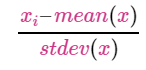1. MinMaxScaler
• Calculate – Subtract min of column & div by the difference between max & min
• Data shifts between 0 & 1
• If distribution not suitable for StandardScaler, this scaler works out.
• Sensitive to outliers.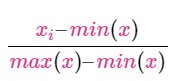1. Robust Scaler
• Suited for data with outliers
• Calculate by subtracting 1st-quartile & div by difference between 3rd-quartile & 1st-quartile.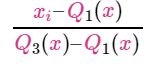1. Normalizer
• Each parameter value is obtained by dividing by magnitude.
• Enabling you to more easily compare data from different places.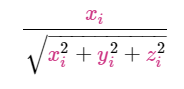1. Binarization
• Thresholding numerical values to binary values ( 0 or 1 )
• A few learning algorithms assume data to be in Bernoulli distribution – Bernoulli’s Naive Bayes
1. Encoding Categorical Value
• Ordinal Values – Low, Medium & High. Relationship between values
• LabelEncoding with the right mapping
1. Imputation
• Missing values cannot be processed by learning algorithms
• Imputers can be used to infer the value of missing details from existing data
1. Polynomial Features
• Deriving non-linear feature by converting information into a higher degree
• Used with linear regression to learn a model of higher degree
1. Custom Transformer
• Often, you will want to convert an existing Python function into a transformer to assist in data cleaning or processing.
• FunctionTransformer is used to create one Transformer
• validate = False, is required for the string column.
1. Text Processing
• Perhaps one of the most common information
• Learning algorithms don’t understand the text but only numbers
• Below methods convert text to numbers
1. CountVectorizer
• Each column represents one word, count refers to the frequency of the word
• A sequence of words is not maintained

13.Hyperparameters

• n_grams – Number of words considered for each column
• stop_words – words not considered
• vocabulary – only words considered
1. TfIdfVectorizer
• Words occurring more frequently in a doc versus entire corpus is considered more important
• The importance is on the scale of 0 & 1
1. HashingVectorizer
• All the above techniques convert information into a table where each word is converted to column
• Learning on data with lakhs of columns is difficult to process
• HashingVectorizer is a useful technique for out-of-core learning
• Multiple words are hashed to limited column
• Limitation – Hashed value to word mapping is not possible
1. Image Processing using skimage
• skimage doesn’t come with anaconda. install with ‘pip install skimage’
• Images should be converted from 0-255 scale to 0-1 scale.
• skimage takes image path & returns numpy array
• images consist of 3 dimensions.